# Trading Using Machine Learning In Python

In recent years, machine learning, more specifically machine learning in Python has become the buzz-word for many quant firms. In their quest to seek the elusive alpha, a number of funds and trading firms have adopted to machine learning. While the algorithms deployed by quant hedge funds are never made public, we know that top funds employ machine learning algorithms to a large extent. Take, for example, Man Group's AHL Dimension programme is a \$5.1 billion dollar hedge fund which is partially managed by AI. There is also Taaffeite Capital which stated that it trades in a fully systematic and automated fashion using proprietary machine learning systems.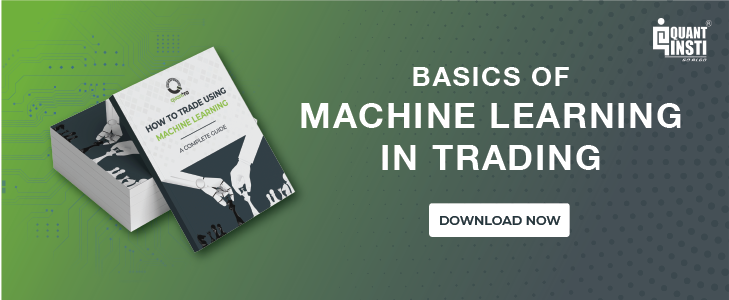In this Python machine learning tutorial, we have tried to understand how machine learning has transformed the world of trading and then we create a simple Python machine learning algorithm to predict the next day’s closing price for a stock. Thus, in this Python machine learning tutorial, we will cover the following topics:

## How machine learning in Python gained popularity

Machine learning packages/libraries are developed in-house by firms for their proprietary use or by third parties who make it freely available to the user community. In recent years, the number of machine learning packages has increased substantially which has helped the developer community in accessing various machine learning techniques and applying the same to their trading needs.

There are hundreds of ML algorithms which can be classified into different types depending on how these work. For example, machine learning regression algorithms are used to model the relationship between variables; decision tree algorithms construct a model of decisions and are used in classification or regression problems. Of these, some algorithms have become popular among quants. Some of these include:

These ML algorithms are used by trading firms for various purposes including:

• Analyzing historical market behaviour using large data sets
• Determine optimal inputs (predictors) to a strategy
• Determining the optimal set of strategy parameters

### But Why Machine Learning in Python?

Over the years, we have realised that Python is becoming a popular language for programmers with that, a generally active and enthusiastic community who are always there to support each other. In fact, as stated in our introductory blog on Python, according to the Developer Survey Results 2019 at stackoverflow, Python is the fastest-growing programming language.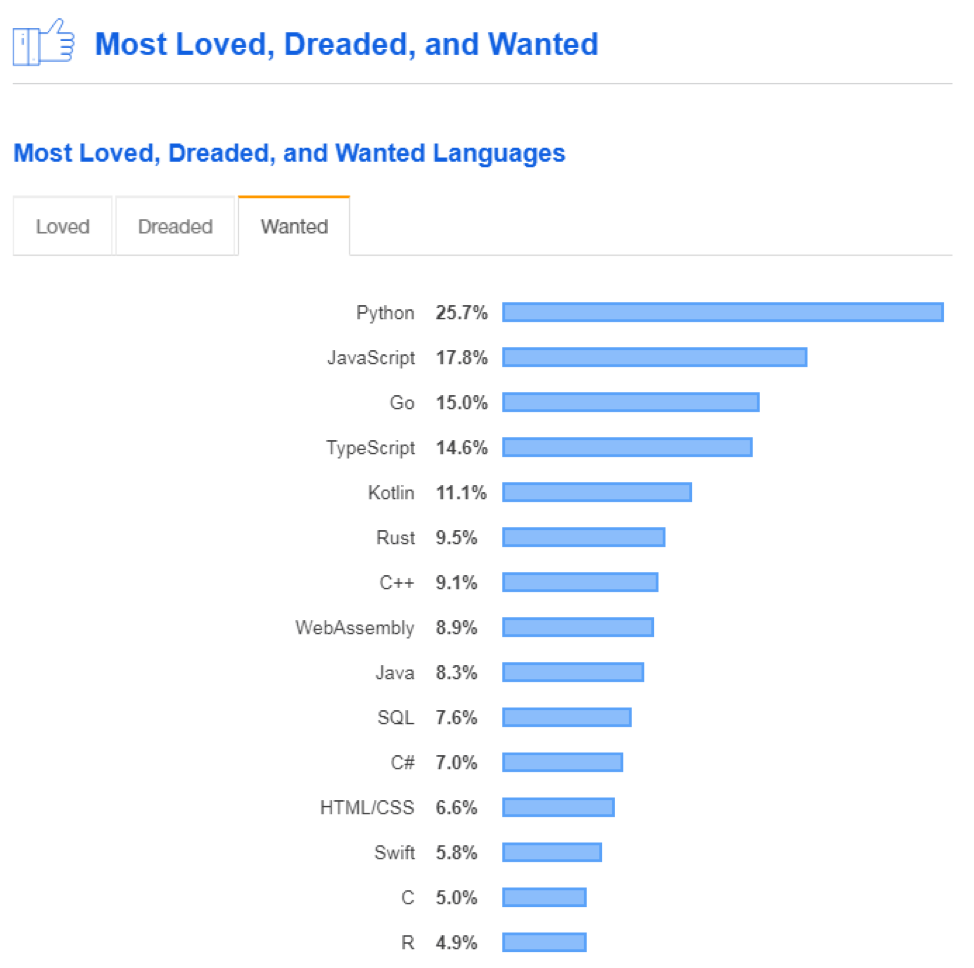It was also found that among the languages the people were most interested to learn, Python was the most desired programming language.

Python trading has gained traction in the quant finance community as it makes it easy to build intricate statistical models with ease due to the availability of sufficient scientific libraries like Pandas, NumPy, PyAlgoTrade, Pybacktest and more. First updates to Python trading libraries are a regular occurrence in the developer community. In fact, Scikit-learn is a Python package developed specifically for machine learning which features various classification, regression and clustering algorithms. Thus, it only makes sense for a beginner (or rather, an established trader themselves), to start out in the world of Python machine learning.

The rise of technology and electronic trading has only accelerated the rate of automated trading in recent years. For a trader or a fund manager, the pertinent question is “How can I apply this new tool to generate more alpha?”. I will explore one such model that answers this question now.

## Pre-requisites for Python machine learning algorithm

You may add one line to install the packages “pip install numpy pandas …” You can install the necessary packages using the following code in the Anaconda Prompt. To know more about Python numpy click here

• pip install pandas
• pip install numpy
• pip install sklearn
• pip install matplotlib

Before we go any further, let me state that this code is written in Python 2.7. So let’s dive in.

### Problem Statement

Let’s start by understanding what we are aiming to do. By the end of this Python machine learning tutorial, I will show you how to create an algorithm that can predict the closing price of a day from the previous OHLC (Open, High, Low, Close) data.

I also want to monitor the prediction error along with the size of the input data.

Let us import all the libraries and packages needed for us to build this machine learning algorithm.

from pandas_datareader import data as web
import numpy as np
from sklearn.linear_model import Lasso
from sklearn.preprocessing import StandardScaler
from sklearn.model_selection import RandomizedSearchCV as rcv
from sklearn.pipeline import Pipeline
from sklearn.preprocessing import Imputer
import matplotlib.pyplot as plt
from IPython import get_ipython

## Getting the data and making it usable

To create any algorithm we need data to train the algorithm and then to make predictions on new unseen data. In this Python machine learning tutorial, we will fetch the data from Yahoo. To accomplish this we will use the data reader function from the panda's library. This function is extensively used and it enables you to get data from many online data sources.

df = web.DataReader('SPY',data_source='yahoo' ,start='2000-01-01',end='2017-03-01')
df=df[['Open','High','Low','Close','Volume']]

We are fetching the data of the SPDR ETF linked to the S&P 500. This stock can be used as a proxy for the performance of the S&P 500 index. We specify the year starting from which we will be pulling the data. Once the data is in, we will discard any data other than the OHLC, such as volume and adjusted Close, to create our data frame ‘df ’.

Now we need to make our predictions from past data, and these past features will aid the machine learning model trade. So, let's create new columns in the data frame that contain data with one day lag.

df['open']=df['Open'].shift(1)
df['high']=df['High'].shift(1)
df['low']=df['Low'].shift(1)
df['close']=df['Close'].shift(1)

Note the capital letters are dropped for lower-case letters in the names of new columns.

## Creating Hyper-parameters

Although the concept of hyper-parameters is worthy of a blog in itself, for now I will just say a few words about them. These are the parameters that the machine learning algorithm can’t learn over but needs to be iterated over. We use them to see which predefined functions or parameters yield the best fit function.

imp = Imputer(missing_values='NaN', strategy='mean', axis=0)

steps = [('imputation', imp),
('scaler',StandardScaler()),
('lasso',Lasso())]

pipeline =Pipeline(steps)

parameters = {'lasso__alpha':np.arange(0.0001,10,.0001),
'lasso__max_iter':np.random.uniform(100,100000,4)}

reg = rcv(pipeline, parameters,cv=5)

In this example, I have used Lasso regression which uses L1 type of regularization. This is a type of machine learning model based on regression analysis which is used to predict continuous data.

This type of regularization is very useful when you are using feature selection. It is capable of reducing the coefficient values to zero. The imputer function replaces any NaN values that can affect our predictions with mean values, as specified in the code. The ‘steps’ is a bunch of functions that are incorporated as a part of the Pipeline function. The pipeline is a very efficient tool to carry out multiple operations on the data set. Here we have also passed the Lasso function parameters along with a list of values that can be iterated over.

Although I am not going into details of what exactly these parameters do, they are something worthy of digging deeper into. Finally, I called the randomized search function for performing the cross-validation.

In this example, we used 5 fold cross-validation. In k-fold cross-validation, the original sample is randomly partitioned into k equal sized subsamples. Of the k subsamples, a single subsample is retained as the validation data for testing the model, and the remaining k-1 subsamples are used as training data.

The cross-validation process is then repeated k times (the folds), with each of the k subsamples used exactly once as the validation data. Cross-validation combines (averages) measures of fit (prediction error) to derive a more accurate estimate of model prediction performance. Based on the fit parameter we decide the best features. In the next section of the Python machine learning tutorial, we will look int test and train sets.

## Splitting the data into test and train sets

First, let us split the data into the input values and the prediction values. Here we pass on the OHLC data with one day lag as the data frame X and the Close values of the current day as y. Note the column names below in lower-case.

X=df[['open','high','low','close']]
y =df['Close']

In this example, to keep the Python machine learning tutorial short and relevant, I have chosen not to create any polynomial features but to use only the raw data. If you are interested in various combinations of the input parameters and with higher degree polynomial features, you are free to transform the data using the PolynomialFeature() function from the preprocessing package of scikit learn.

Now, let us also create a dictionary that holds the size of the train data set and its corresponding average prediction error.

avg_err={}

## Getting the best-fit parameters to create a new function

I want to measure the performance of the regression function as compared to the size of the input dataset. In other words, I want to see if by increasing the input data, will we be able to reduce the error. For this, I used for loop to iterate over the same data set but with different lengths.

At this point, I would like to add that for those of you who are interested, explore the ‘reset’ function and how it will help us in making a more reliable prediction.

(Hint: It is a part of the Python magic commands)

for t in np.arange(50,97,3):
get_ipython().magic('reset_selective -f reg1')
split = int(t*len(X)/100)
reg.fit(X[:split],y[:split])
best_alpha = reg.best_params_['lasso__alpha']
best_iter= reg.best_params_['lasso__max_iter']
reg1= Lasso(alpha=best_alpha,max_iter=best_iter)
X=imp.fit_transform(X,y)
reg1.fit(X[:split],y[:split])


Let me explain what I did in a few steps.

First, I created a set of periodic numbers ‘t’ starting from 50 to 97, in steps of 3. The purpose of these numbers is to choose the percentage size of the dataset that will be used as the train data set.

Second, for a given value of ‘t’, I split the length of the data set to the nearest integer corresponding to this percentage. Then I divided the total data into train data, which includes the data from the beginning till the split, and test data, which includes the data from the split till the end. The reason for adopting this approach and not using the random split is to maintain the continuity of the time series.

After this, we pull the best parameters that generated the lowest cross-validation error and then use these parameters to create a new reg1 function which will be a simple Lasso regression fit with the best parameters.

## Making the predictions and checking the performance

Now let us predict the future close values. To do this we pass on test X, containing data from split to end, to the regression function using the predict() function. We also want to see how well the function has performed, so let us save these values in a new column.

    df['P_C_%i'%t]=0.
df.iloc[:,df.columns.get_loc('P_C_%i'%t)]=reg1.predict(X[:])
df['Error_%i'%t]= np.abs(df['P_C_%i'%t]-df['Close'])

e =np.mean(df['Error_%i'%t][split:])
train_e= np.mean(df['Error_%i'%t][:split])
avg_err[t]=e
avg_train_err[t]=train_e


As you might have noticed, I created a new error column to save the absolute error values. Then I took the mean of the absolute error values, which I saved in the dictionary that we had created earlier.

Now it's time to plot and see what we got.

Range =df['high'][split:]-df['low'][split:]
plt.scatter(list(avg_train_err.keys()),list(avg_train_err.values()),label='train_error')
plt.legend(loc='best')
print ('\nAverage Range of the Day:',np.average(Range))


I created a new Range value to hold the average daily trading range of the data. It is a metric that I would like to compare with when I am making a prediction. The logic behind this comparison is that if my prediction error is more than the day’s range then it is likely that it will not be useful.

I might as well use the previous day’s High or Low as the prediction, which will turn out to be more accurate. Please note I have used the split value outside the loop. This implies that the average range of the day that you see here is relevant to the last iteration.

Let’s execute the code and see what we get.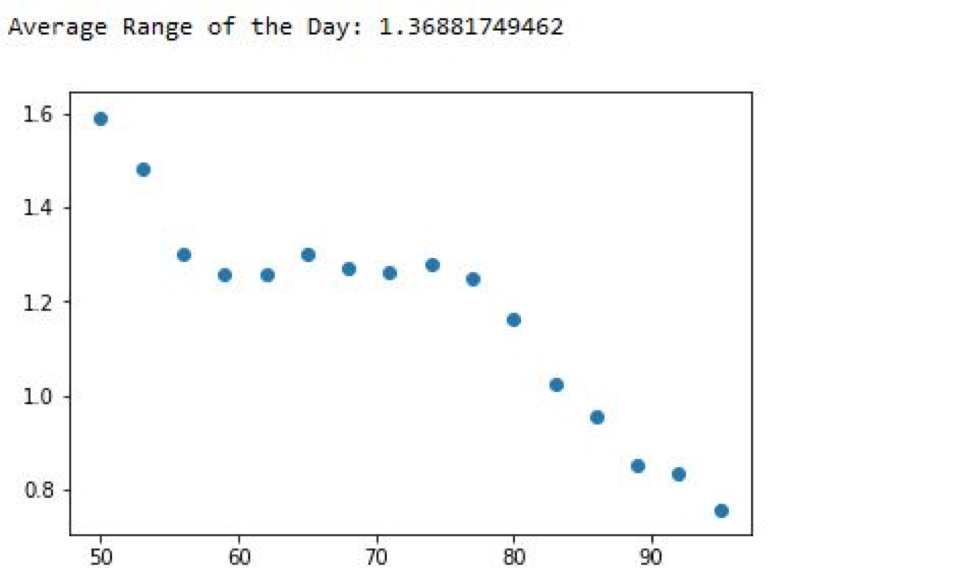Some food for thought

What does this scatter plot tell you? Let me ask you a few questions.

• Is the equation over-fitting?
• The performance of the data improved remarkably as the train data set size increased. Does this mean if we give more data the error will reduce further?
• Is there an inherent trend in the market, allowing us to make better predictions as the data set size increases?
• Last but the best question How will we use these predictions to create a trading strategy?

## Bonus: FAQ related to the Python Machine Learning Algorithm

At the end of the last section of the Python machine learning tutorial, I asked a few questions. Now, I will answer them all at the same time. I will also discuss a way to detect the regime/trend in the market without training the algorithm for trends.

But before we go ahead, please use a fix to fetch the data from Google to run the code below.

from pandas_datareader import data as web
import numpy as np
from sklearn.linear_model import Lasso
from sklearn.preprocessing import StandardScaler
from sklearn.model_selection import RandomizedSearchCV as rcv
from sklearn.pipeline import Pipeline
from sklearn.preprocessing import Imputer
import matplotlib.pyplot as plt
from IPython import get_ipython

avg_err={}
avg_train_err={}


#### If you face challenges while downloading the market data from Yahoo and Google Finance platforms and are looking for an alternative source for market data, you can use Quandl for the same.

import quandl
from datetime import datetime
data = quandl.get('EOD/AAPL', start_date='2017-1-1', end_date='2018-1-1', api_key= api_key)
# Note that you need to know the "Quandl code" of each dataset you download.
# In the above example, it is 'EOD/AAPL'.
#Then, you can find your API key on Quandl account settings page.

Is the equation over-fitting?

This was the first question I had asked. To know if your data is overfitting or not, the best way to test it would be to check the prediction error that the algorithm makes in the train and test data.

To do this, we will have to add a small piece of code to the already written code.

from pandas_datareader import data as web
import numpy as np
from sklearn.linear_model import Lasso
from sklearn.preprocessing import StandardScaler
from sklearn.model_selection import RandomizedSearchCV as rcv
from sklearn.pipeline import Pipeline
from sklearn.preprocessing import Imputer
import matplotlib.pyplot as plt
from IPython import get_ipython

avg_err={}
avg_train_err={}
df=df[['Open','High','Low','Close','Volume']]
df['open']=df['Open'].shift(1)
df['high']=df['High'].shift(1)
df['low']=df['Low'].shift(1)
df['close']=df['Close'].shift(1)
df['volume']=df['Volume'].shift(1)

X=df[['open','high','low','close']]
y =df['Close']

imp = Imputer(missing_values='NaN', strategy='mean', axis=0)

steps = [('imputation', imp),
('scaler',StandardScaler()),
('lasso',Lasso())]

pipeline =Pipeline(steps)

parameters = {'lasso__alpha':np.arange(0.0001,10,.0001),
'lasso__max_iter':np.random.uniform(100,100000,4)}

reg = rcv(pipeline, parameters,cv=5)

for t in np.arange(50,97,3):
get_ipython().magic('reset_selective -f reg1')
split = int(t*len(X)/100)
reg.fit(X[:split],y[:split])
best_alpha = reg.best_params_['lasso__alpha']
best_iter= reg.best_params_['lasso__max_iter']
reg1= Lasso(alpha=best_alpha,max_iter=best_iter)
X=imp.fit_transform(X,y)
reg1.fit(X[:split],y[:split])

df['P_C_%i'%t]=0.
df.iloc[:,df.columns.get_loc('P_C_%i'%t)]=reg1.predict(X[:])
df['Error_%i'%t]= np.abs(df['P_C_%i'%t]-df['Close'])

e =np.mean(df['Error_%i'%t][split:])
train_e= np.mean(df['Error_%i'%t][:split])
avg_err[t]=e
avg_train_err[t]=train_e
Range =df['high'][split:]-df['low'][split:]

plt.scatter(list(avg_err.keys()),list(avg_err.values()), label='test_error')
plt.scatter(list(avg_train_err.keys()),list(avg_train_err.values()),label='train_error')
plt.legend(loc='best')
print ('\nAverage Range of the Day:',np.average(Range))


First, let me begin my explanation by apologizing for breaking the norms: going beyond the 80 column mark.

Second, if we run this piece of code, then the output would look something like this.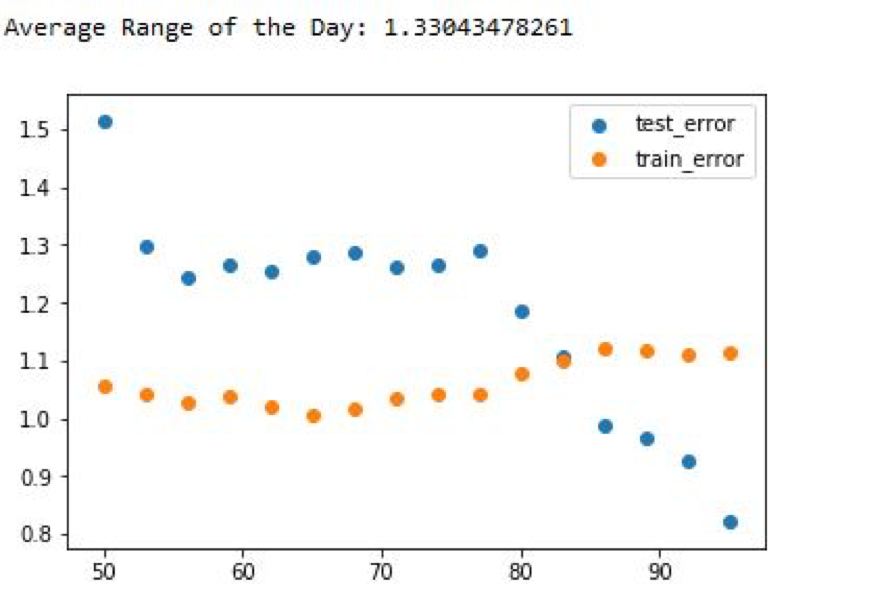Our algorithm is doing better in the test data compared to the train data. This observation in itself is a red flag. There are a few reasons why our test data error could be better than the train data error:

1. If the train data had greater volatility (Daily range) compared to the test set, then the prediction would also exhibit greater volatility.
2. If there was an inherent trend in the market that helped the algo make better predictions.

Now, let us check which of these cases is true. If the range of the test data was less than the train data, then the error should have decreased after passing more than 80% of the data as a train set, but it increases.

Next, to check if there was a trend, let us pass more data from a different time period.

dfre
from pandas_datareader import data as web
import numpy as np
import pandas as pd
from sklearn import mixture as mix
import seaborn as sns
import matplotlib.pyplot as plt
01're)


If we run the code the result would look like this: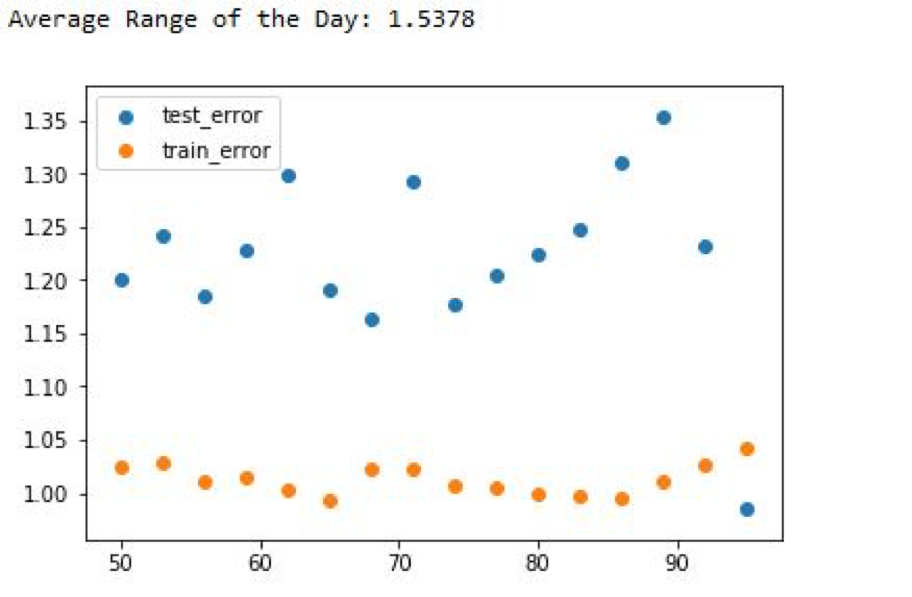So, giving more data did not make your algorithm works better, but it made it worse. In time-series data, the inherent trend plays a very important role in the performance of the algorithm on the test data. As we saw above it can yield better than expected results sometimes. The main reason why our algo was doing so well was the test data was sticking to the main pattern observed in the train data.

So, if our algorithm can detect underlying the trend and use a strategy for that trend, then it should give better results. I will explain this in more detail:

• Can the machine learning algorithm detect the inherent trend or market phase (bull/bear/sideways/breakout/panic)?
• Can the database be trimmed in a way to train different algos for different situations

The answer to both the questions is YES!

We can divide the market into different regimes and then use these signals to trim the data and train different algorithms for these datasets. To achieve this, I choose to use an unsupervised machine learning algorithm.

From here on, this Python machine learning tutorial will be dedicated to creating an algorithm that can detect the inherent trend in the market without explicitly training for it.

First, let us import the necessary libraries.

from pandas_datareader import data as web
import numpy as np
import pandas as pd
from sklearn import mixture as mix
import seaborn as sns
import matplotlib.pyplot as plt


Then we fetch the OHLC data from Google and shift it by one day to train the algorithm only on the past data.

df= web.get_data_yahoo('SPY',start= '2000-01-01', end='2017-01-01')
df=df[['Open','High','Low','Close']]
df['open']=df['Open'].shift(1)
df['high']=df['High'].shift(1)
df['low']=df['Low'].shift(1)
df['close']=df['Close'].shift(1)

df=df[['open','high','low','close']]


Then drop all the NaN.

df=df.dropna()

Next, we will instantiate an unsupervised machine learning algorithm using the ‘Gaussian mixture’ model from sklearn.

unsup = mix.GaussianMixture(n_components=4,
covariance_type="spherical",
n_init=100,
random_state=42)


In the above code, I created an unsupervised-algo that will divide the market into 4 regimes, based on the criterion of its own choosing. We have not provided any train dataset with labels like in the previous section of the Python machine learning tutorial.

Next, we will fit the data and predict the regimes. Then we will be storing these regime predictions in a new variable called regime.

unsup.fit(np.reshape(df,(-1,df.shape)))
regime = unsup.predict(np.reshape(df,(-1,df.shape)))


Now let us calculate the returns of the day.

df['Return']= np.log(df['close']/df['close'].shift(1))


Then, create a dataframe called Regimes which will have the OHLC and Return values along with the corresponding regime classification.

Regimes=pd.DataFrame(regime,columns=['Regime'],index=df.index)\
.join(df, how='inner')\
.assign(market_cu_return=df.Return.cumsum())\
.reset_index(drop=False)\
.rename(columns={'index':'Date'})


After this, let us create a list called ‘order’ that has the values corresponding to the regime classification, and then plot these values to see how well the algo has classified.

order=[0,1,2,3]
fig = sns.FacetGrid(data=Regimes,hue='Regime',hue_order=order,aspect=2,size= 4)
plt.show()


The final regime differentiation would look like this: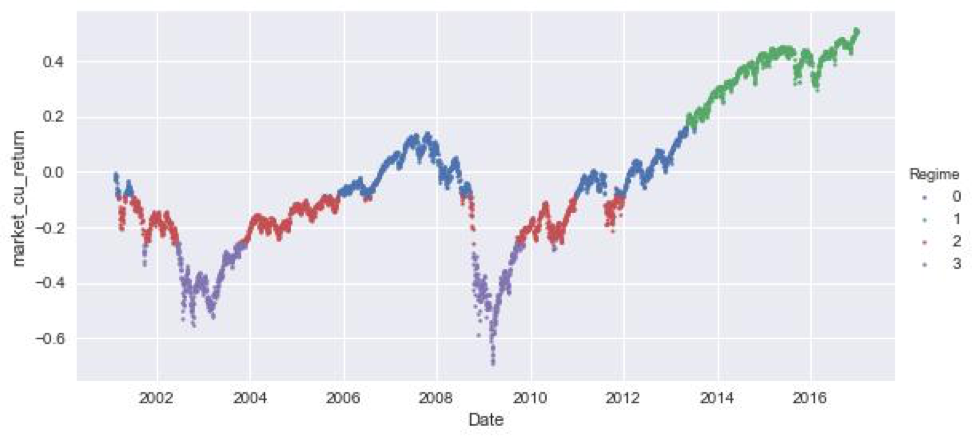This graph looks pretty good to me. Without actually looking at the factors based on which the classification was done, we can conclude a few things just by looking at the chart.

1. The red zone is the low volatility or the sideways zone
2. The purple zone is high volatility zone or panic zone.
3. The green zone is a breakout zone.
4. The blue zone: Not entirely sure but let us find out.

Use the code below to print the relevant data for each regime.

for i in order:
print('Mean for regime %i: '%i,unsup.means_[i])
print('Co-Variancefor regime %i: '%i,(unsup.covariances_[i]))


The output would look like this: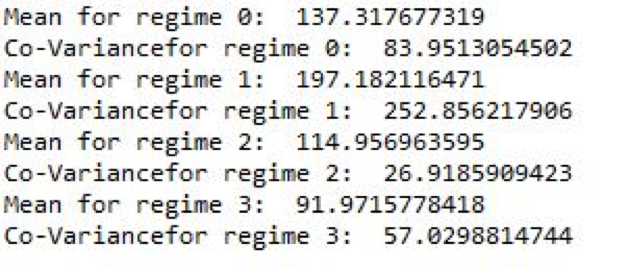The data can be inferred as follows:

1. Regime 0: Low mean and High covariance.
2. Regime 1: High mean and High covariance.
3. Regime 2: High mean and Low covariance.
4. Regime 3: Low mean and Low covariance.

So far, we have seen how we can split the market into various regimes.

But the question of implementing a successful strategy is still unanswered. If you want to learn how to code a machine learning trading strategy then your choice is simple:

To rephrase Morpheus,

This is your last chance. After this, there is no turning back. You take the blue pill—the story ends, you wake up in your bed and believe that you can trade manually. You take the red pill—you stay in the Algoland, and I show you how deep the rabbit hole goes.

Remember: all I'm offering is the truth. Nothing more.

## A step further in the world of Machine Learning

Keeping oneself updated is of prime importance in today’s world. Having a learner’s mindset always helps to enhance your career and picking up skills and additional tools in the development of trading strategies for themselves or their firms.

Here are a few books which might be interesting:

1. Gareth James, Daniela Witten, Trevor Hastie and Robert Tibshirani Introduction to statistical learning
2. The Hundred-Page Machine Learning Book by Andriy Burkov
3. Hastie, Tibshirani, and Friedman's The Elements of Statistical Learning

## Machine Learning Competitions

There are a number of sites which host ML competitions. These competitions although not specifically targeted towards the application of Python machine learning in trading, can give good exposure to quants and traders to different ML problems via participation in competitions & forums and help expand their ML knowledge. Some of the popular ML competition hosting sites include:

Sign up for our latest course on ‘Decision Trees in Trading‘ on Quantra. This course is authored by Dr. Ernest P. Chan and demystifies the black box within classification trees, helps you to create trading strategies and will teach you to understand the limitations of your models. This course consists of 7 sections from basic to advanced topics.

Disclaimer: All data and information provided in this article are for informational purposes only. QuantInsti® makes no representations as to accuracy, completeness, currentness, suitability, or validity of any information in this article and will not be liable for any errors, omissions, or delays in this information or any losses, injuries, or damages arising from its display or use. All information is provided on an as-is basis.